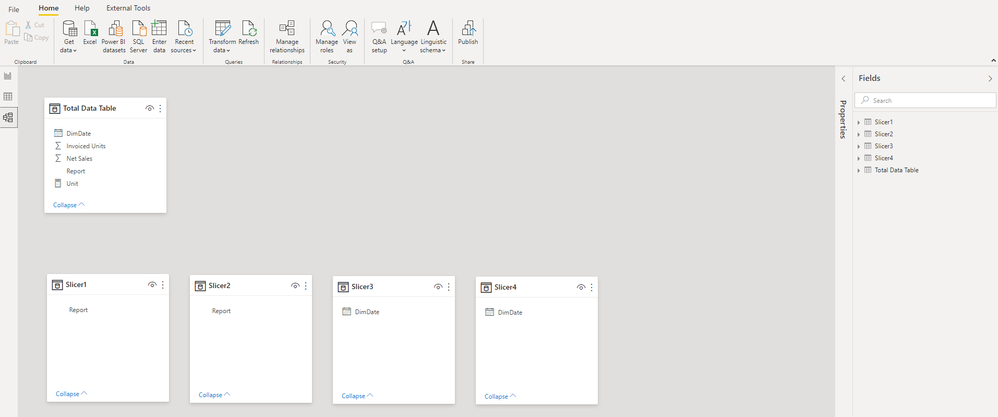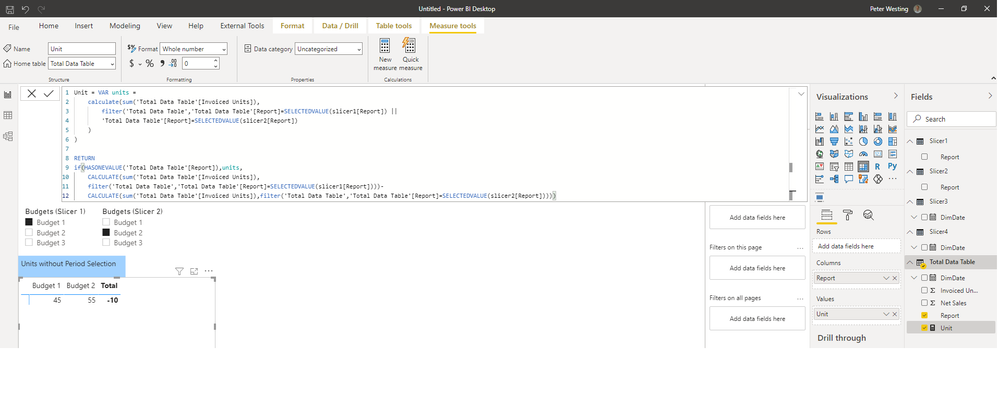cancel
Showing results for
Did you mean:

Fabric is Generally Available. Browse Fabric Presentations. Work towards your Fabric certification with the Cloud Skills Challenge.Anonymous
Not applicable

## Budget Variance - Multiple Slicers

Main Scope

The main idea is to create a price variance between the selected reports, within the selected time frames.

The user of the dashboard will:

1) Select the current budget and period

2) Select the comparing budget and period

Data Setup

The sample dataset contains of the following data:

The Main Data:

- Reports (Categorical variable for the different budget)

- DimDate (Date of expected invoicing)

- Net Sales

- Units

Besides, I created two seperate tables that are not connected to the Main Data:

Slicer1 = VALUES('Main Data'[Report])
Slicer2 = VALUES('Main Data'[Report])Current Problem:
To create a measure for price variance I will need to create a measure for units and net sales that
1) filters for the selected budget and period in the current period
2) filters the selected budget and period in the comparing period
3) gives the variance of the above selections

Initially, I created a measure that filters only the selected budgets based on the selections of the budgets:
Unit = VAR units =
calculate(sum('Total Data Table'[Invoiced Units]),
filter('Total Data Table','Total Data Table'[Report]=SELECTEDVALUE(slicer1[Report]) ||
'Total Data Table'[Report]=SELECTEDVALUE(slicer2[Report])))

RETURN
if(HASONEVALUE('Total Data Table'[Report]),units,
CALCULATE(sum('Total Data Table'[Invoiced Units]), filter('Total Data Table','Total Data Table'[Report]=SELECTEDVALUE(slicer1[Report])))-
CALCULATE(sum('Total Data Table'[Invoiced Units]),filter('Total Data Table','Total Data Table'[Report]=SELECTEDVALUE(slicer2[Report]))))This formula does not filter for the selected period, only the selected budgets.
I would like to compare different budgets accross different periods.
For Example:
- Compare Budget 1 in period 01/01/2019 - 12/03/2019
- To Budget 2 in period 01/07/2019 - 12/09/2019I have tried multiple ways to do this, but have not found any that worked; I hope a smart solutions exists to this issue.

1 ACCEPTED SOLUTIONSuper User

@Anonymous , Please refer these measures set 1- Slicer 1, Sclicer 3 and Set 2 - Slicer 2 and Slicer 4

Set 1 =
var _max = maxx(allselected(Slicer3), Slicer3[Date])
var _min = minx(allselected(Slicer3), Slicer3[Date])
return
CALCULATE(sum('Total Data Table'[Invoiced Units]), filter('Total Data Table','Total Data Table'[Report] in allselected(slicer1[Report])
&& 'Total Data Table'[Dimdate] >=_min && 'Total Data Table'[Dimdate] <=_max))

Set 2 =
var _max = maxx(allselected(Slicer4), Slicer4[Date])
var _min = minx(allselected(Slicer4), Slicer4[Date])
return
CALCULATE(sum('Total Data Table'[Invoiced Units]), filter('Total Data Table','Total Data Table'[Report] in allselected(slicer2[Report])
&& 'Total Data Table'[Dimdate] >=_min && 'Total Data Table'[Dimdate] <=_max))

2 REPLIES 2Super User

@Anonymous , Please refer these measures set 1- Slicer 1, Sclicer 3 and Set 2 - Slicer 2 and Slicer 4

Set 1 =
var _max = maxx(allselected(Slicer3), Slicer3[Date])
var _min = minx(allselected(Slicer3), Slicer3[Date])
return
CALCULATE(sum('Total Data Table'[Invoiced Units]), filter('Total Data Table','Total Data Table'[Report] in allselected(slicer1[Report])
&& 'Total Data Table'[Dimdate] >=_min && 'Total Data Table'[Dimdate] <=_max))

Set 2 =
var _max = maxx(allselected(Slicer4), Slicer4[Date])
var _min = minx(allselected(Slicer4), Slicer4[Date])
return
CALCULATE(sum('Total Data Table'[Invoiced Units]), filter('Total Data Table','Total Data Table'[Report] in allselected(slicer2[Report])
&& 'Total Data Table'[Dimdate] >=_min && 'Total Data Table'[Dimdate] <=_max))Anonymous
Not applicable

@amitchandak Thank you, this helped me out a lot! I created the two sets within a measure with the following formula:

Total Units =
var set1_max = maxx(allselected(Slicer3), Slicer3[Date])
var set1_min = minx(allselected(Slicer3), Slicer3[Date])
VAR units_set1 = CALCULATE(sum('Total Data Table'[Invoiced Units]), filter('Total Data Table','Total Data Table'[Report] in allselected(slicer1[Report])
&& 'Total Data Table'[Date] >= set1_min && 'Total Data Table'[Date] <= set1_max))

var set2_max = maxx(allselected(Slicer4), Slicer4[Date])
var set2_min = minx(allselected(Slicer4), Slicer4[Date])
VAR units_set2 = CALCULATE(sum('Total Data Table'[Invoiced Units]), filter('Total Data Table','Total Data Table'[Report] in allselected(slicer2[Report])
&& 'Total Data Table'[Date] >= set2_min && 'Total Data Table'[Date] <= set2_max))

VAR Totalunits = calculate(sum('Total Data Table'[Invoiced Units]),FILTER('Total Data Table','Total Data Table'[Report] in allselected(slicer2[Report])
&& 'Total Data Table'[Date] >= set2_min && 'Total Data Table'[Date] <= set2_max ||
'Total Data Table'[Report] in allselected(slicer1[Report])
&& 'Total Data Table'[Date] >= set1_min && 'Total Data Table'[Date] <= set1_max))

RETURN
if(HASONEVALUE('Total Data Table'[Report]),Totalunits,units_set1-units_set2)

If I put the amount of units in a table for the selected budget and the selected time periods. Besides, it gives the variance between them.It is a beautiful dax code. Again, thank you very much!Announcements#### Power BI Monthly Update - November 2023

Check out the November 2023 Power BI update to learn about new features.#### Fabric Community News unified experience

Read the latest Fabric Community announcements, including updates on Power BI, Synapse, Data Factory and Data Activator.#### The largest Power BI and Fabric virtual conference

130+ sessions, 130+ speakers, Product managers, MVPs, and experts. All about Power BI and Fabric. Attend online or watch the recordings.Top Solution Authors
Top Kudoed Authors
Users online (4,142)